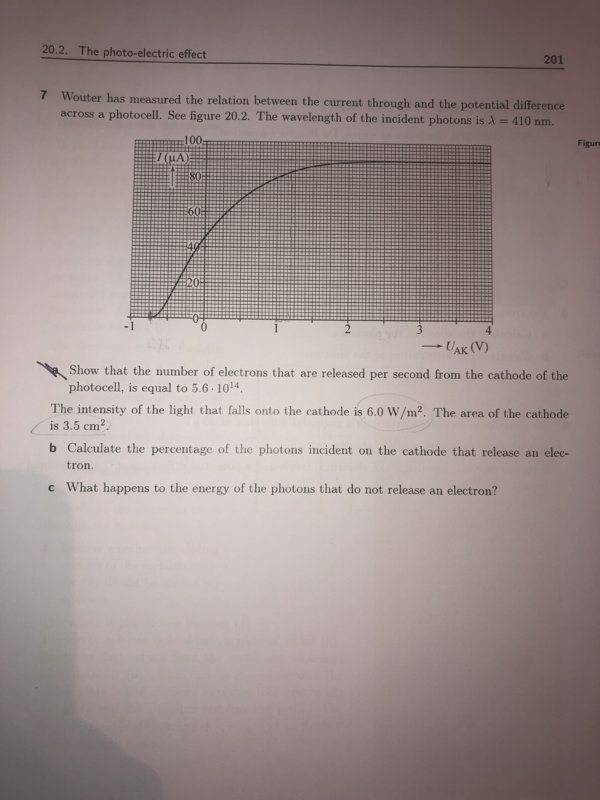# Photoelectric effect question

• Zeynaz

#### Zeynaz

Homework Statement
The intensity of the light that falls onto the cathode is 6.0 W/m2. The area of the cathode of the photocell is 3.5 cm^2

b) Calculate the percentage of the photons incident on the cathode that release an electron.

c) what happens to the energy of the photons that do not release an electron
Relevant Equations
E=hf
V=IR
P=IV
I=n(q-electron)The full questions is in the picture. I already solved a) and found 5.6E14 electrons per second

For b) i first found the power of the light but just multiplying the intensity with the area: (6.0 W/m2)(3.5E-4 m^2) = 0.0021 W
Then I tried to use the voltage from the graph but i am not sure which value i should use because i don't know the voltage that corresponds to the intensity given.

So could you show me a way that can help me work it out?

After finding the number of electrons that the light releases i would calculate the percetage by dividing the value in question a) by the value i find and multiply it with 100.
(The correct answer should be 13% but i don't know how to get there)

For c) i said that because the energy of the photons are not enough to eject the electrons on the plate are a reflected back. (But I am not sure if that's a correct statement)

i am not sure which value i should use because i don't know the voltage that corresponds to the intensity given.

I don't think they're related. The intensity is a property of the incoming light, and it's the same light source with the same intensity no matter what you're doing with the photocell, or even if you don't have a photocell, you just replace it with a rock.

That's just telling you how much energy is incident from the outside light source.

A photon releases an electron, by being absorbed. Since you know how many electrons are released (each second) then you know how many photons were absorbed each second. The question is asking what percentage that is, of the photons which were incident in that same second.

For b) i first found the power of the light but just multiplying the intensity with the area: (6.0 W/m2)(3.5E-4 m^2) = 0.0021 W
Then I tried to use the voltage from the graph but i am not sure which value i should use because i don't know the voltage that corresponds to the intensity given.

So could you show me a way that can help me work it out?

After multiplying with area of plate you found the power transferred to the plate. Now power is energy transferred per second. So of course you need to find the energy of each photon first.

For part c, the photons which don't eject electrons can be absorbed by the material of the plate to generate heat, or they can be reflected, scattered etc. Energy is conserved.